# R Data Generator

Contents[Hide]

The R Data Generator transform lets you generate data by writing scripts using the R statistical programming language. R is both a programming language and an environment for statistical computing, graphics, and predictive analysis.

You can use the R Data Generator transform to generate data for prototyping or developing proof-of-concept dashboards.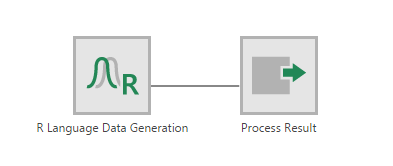Transform - R Data Generator

To learn more about the R language, see The R Project for Statistical Computing.

## 1. Setup

Before you can use the R Data Generator transform in Dundas BI, the R programming environment must be installed on a server.

See Install and configure R for more details.

## 2. Input

The R Data Generator transform does not have any inputs. It just generates output by running R scripts against the R server.

## 3. Add the transform

When creating a new data cube, you can add the R Data Generator transform to an empty canvas from the toolbar.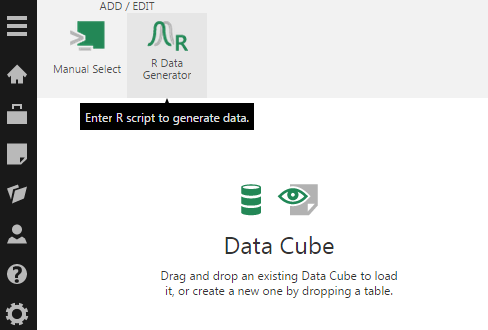Add the R Data Generator transform from the toolbar

The R Language Data Generation transform is added to the data cube and connected to a Process Result transform automatically.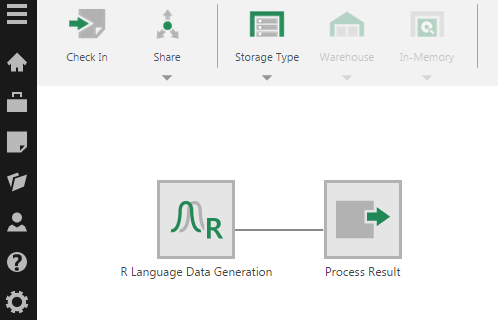The R Language Data Generation transform is added

You can also add the R Data Generator transform from the toolbar to an existing data cube process. A typical example is to connect the R Language Data Generation instance to a Union transform which merges data from multiple inputs.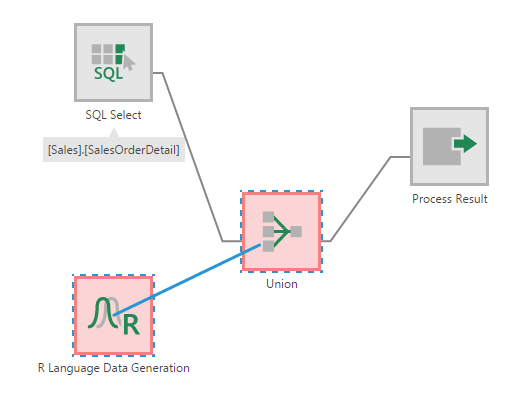Merging R Data Generator output with other data using a Union transform

## 4. Configure the transform

Double click the R Language Data Generation transform or select the Configure option from its right-click menu.

In the configuration dialog for the transform, the key task is to enter an R script that sets the output variable.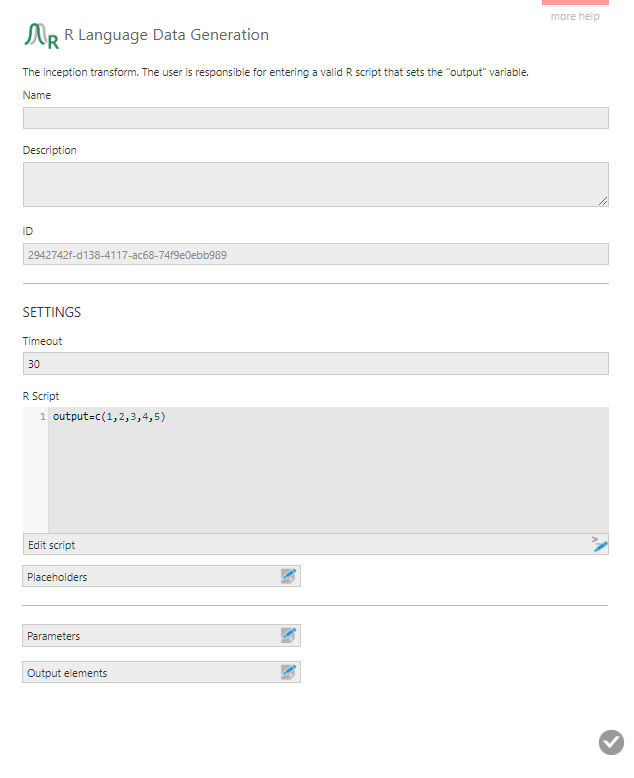Configure the transform by entering an R script that sets the output variable

For example, a simple script for generating a column of numbers from 1 to 5 looks like this:

`output=c(1,2,3,4,5)`

In this dialog, you can set up Placeholders to insert into the script that pass in parameter values similar to when using a manual select.

You can also set up Parameters to directly filter this transform's output like with select transforms.

## 5. Output

The output of the R Data Generator depends on the R script it is configured with. It can be a single value, a column of values, or multiple columns.

In the case of the simple script for generating numbers from 1 to 5, you can see an output column named 'Data' by selecting the Process Result transform and then clicking on Data Preview.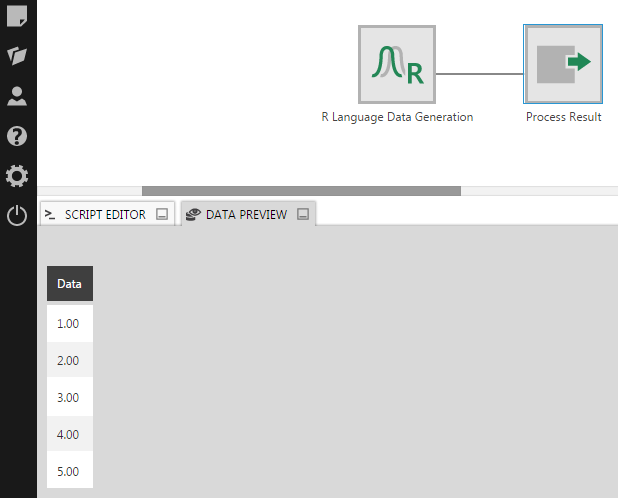Data Preview for R Data Generator output

## 6. Example R scripts

Here are some example R scripts for generating data.

### 6.1. Random number generation

Generate 10 random numbers between 200.5 and 300.5:

`output=runif(10, 200.5, 300.5)`

Generate 5 random integers between 1 and 1000:

`output=sample(1:1000, 5)`

Generate two columns of data. The first column contains integers from 1 to 5 in order. The second column contains 5 random integers between 50 and 100:

```x=c(1,2,3,4,5)
y=sample(50:100, 5)
output=data.frame(x,y)```

Generate two columns, the first column with 12 random dates between 2017/01/01 and 2018/01/01, and the 2nd column with 12 random integers between 1 and 1000 :

```x=sample(1:1000, 12)
y=sample(seq(as.Date('2017/01/01'), as.Date('2018/01/01'), by="day"), 12)
output=data.frame(x,y)```

### 6.2. Pre-defined datasets

Load pre-defined data from the R Datasets Package. For example, Freeny's Revenue Data:

`output=datasets::freeny`

Here's the resulting Data Preview: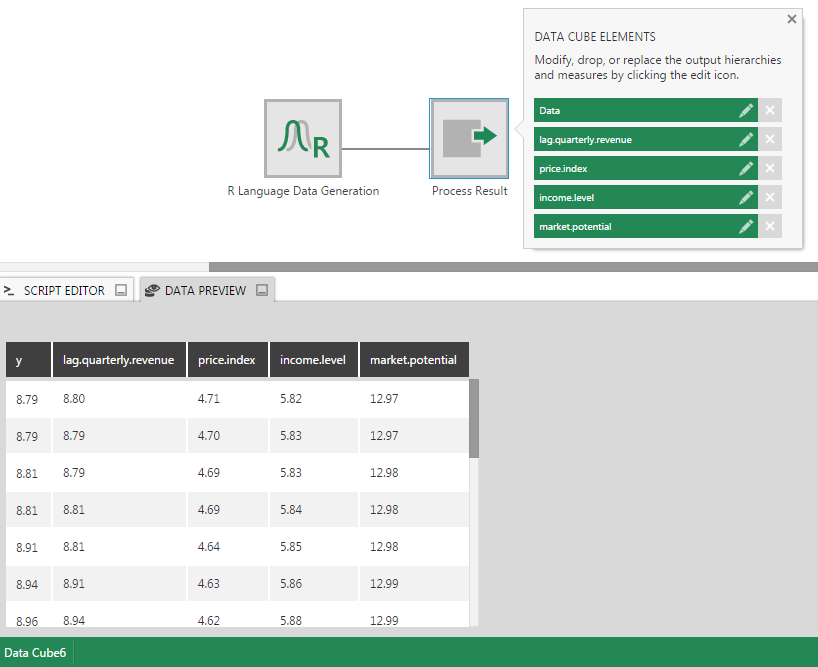Using a pre-defined R dataset

## 7. See also

Dundas Data Visualization, Inc.
500-250 Ferrand Drive
Toronto, ON, Canada
M3C 3G8

North America: 1.800.463.1492
International: 1.416.467.5100

Dundas Support Hours:
Phone: 9am-6pm, ET, Mon-Fri
Email: 7am-6pm, ET, Mon-Fri